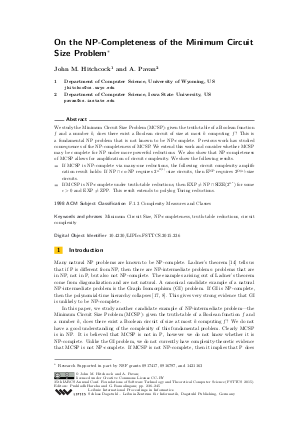Document# On the NP-Completeness of the Minimum Circuit Size Problem

### Authors John M. Hitchcock, A. Pavan## File

LIPIcs.FSTTCS.2015.236.pdf
• Filesize: 399 kB
• 10 pages

## Cite As

John M. Hitchcock and A. Pavan. On the NP-Completeness of the Minimum Circuit Size Problem. In 35th IARCS Annual Conference on Foundations of Software Technology and Theoretical Computer Science (FSTTCS 2015). Leibniz International Proceedings in Informatics (LIPIcs), Volume 45, pp. 236-245, Schloss Dagstuhl - Leibniz-Zentrum für Informatik (2015)
https://doi.org/10.4230/LIPIcs.FSTTCS.2015.236

## Abstract

We study the Minimum Circuit Size Problem (MCSP): given the truth-table of a Boolean function f and a number k, does there exist a Boolean circuit of size at most k computing f? This is a fundamental NP problem that is not known to be NP-complete. Previous work has studied consequences of the NP-completeness of MCSP. We extend this work and consider whether MCSP may be complete for NP under more powerful reductions. We also show that NP-completeness of MCSP allows for amplification of circuit complexity. We show the following results. - If MCSP is NP-complete via many-one reductions, the following circuit complexity amplification result holds: If NP cap co-NP requires 2^n^{Omega(1)-size circuits, then E^NP requires 2^Omega(n)-size circuits. - If MCSP is NP-complete under truth-table reductions, then EXP neq NP cap SIZE(2^n^epsilon) for some epsilon> 0 and EXP neq ZPP. This result extends to polylog Turing reductions.
##### Keywords
• Minimum Circuit Size
• NP-completeness
• truth-table reductions
• circuit complexity

## Metrics

• Access Statistics
• Total Accesses (updated on a weekly basis)
0